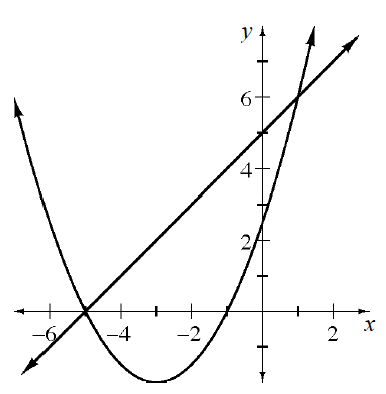### Home > A2C > Chapter 5 > Lesson 5.2.4 > Problem5-97

5-97.

Consider the graph at right as you answer the following questions.

1. Find the equation of the parabola.

Look for $x$-intercepts and vertex coordinates.

$x$-intercepts: $x=−1$ and $x=−5$

vertex: $(−3,−2)$

2. Find the equation of the line.

3. Use your graph to solve $x+5=\frac{1}{2}(x+3)^2−2$.

$x=−5$ or $x=1$

4. Use your graph to solve the system:
$y=\frac{1}{2}(x+3)^2−2$
$y=x+5$

5. Use your graph to solve the inequality $x+5<\frac{1}{2}(x+3)^2−2$.

$x<−5$ or $x>1$

6. Use your graph to solve $\frac{1}{2}(x+3)^2−2=0$.

$x=−5$ or $x=−1$

7. Use your graph to solve $x+5=4$.

Add the line $y=4$. Where does it intersect the line
$y=x+5$?

$x=−1$

8. How could you change the equation of the parabola so that the parabola and the line do not intersect? Is there more than one way?

What can you do to the equation of the parabola to change its position on the graph?
How could you move it up? Or flip it over?